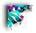# Thermodynamics in "Real state"

Notes on usage of the Thermodynamics tutorial

The Java program above has three panels. This is the same program we used in the kinetics pages, but here with a different purpose. Instead of concentrating on the enzyme kinetics this time..

2. please slide the kcat/KM slider about halfway up to make the concentrations change at an appreciable rate.
3. Now grab the "DG" slider and move. This directly controls a pair of bar graphs in the upper right. The equilibrium position for your reaction.

Immediately to the left of this are three bars. The current [S], current [P] and observed rate of conversion (red bar).

## What to look for.

1. Set the DG to some value
2. Watch [S] and [P] change until it reaches the same ratio as the red bars in the upper right
3. The rate bar starts high and decreases (NOTE: THIS IS A NET RATE OF CONVERSION OF S TO P)
4. You should see that when the current [S] and [P] are at the same ratio as the red bars the NET rate of conversion is 0
5. This means that the forward rate = reverse rate.
6. Increase the [P] slider. and observe what happens to [S]. note; if you start with [P] very high the observed rate is negative (S is forming faster than P)

### Notes

• The top depicts a graph of the Enzyme rate vs. [S]. There are two lines on the first graph here:
• Black: is a reference graph whose Data (Km and Vmax) ALWAYS remain unchanged even as the scale on the graph changes.
• RED represents the data that you manipulate with the sliders.
• A relative scale of the graph is indicated at the top.
• The two bar graphs on the top right represent formation about the current conditions chosen
• The panel at top right gives a bar graph representation of the current [S], [P], and enzyme rates as well as
• The log of the final equilibrium position.
• The middle panel provides numerical representation of the same data.
• units for the parameters
• all concentrations ([S], [P], [E]) are in M/l (Molar)
• kcat = sec-1
• enzyme rates (vmax and vo=Rate of [P]) = moles/sec (moles of product produced per sec)
• Km = Molar
• kcat/Km = Molar sec
• Keq is unitless
• The bottom panel provides some interaction.

• Sliders on the bottom panel allow you to independently alter the [S], [P], [E], kcat, Km, Vmax, kcat/Km, Keq and the Hill coefficient independently to observe the effects.
• The Radio buttons on the right allow you to maintain the [P]= 0 or allow [P] to accumulate.

In the latter case you should observe that eventually the ratio of [S] and [P] will come to the same ratio as indicated in the "final equilibrium position" window. This final equilibrium position can be altered by changing the DG of the reaction.

The bar graphs update in at a rate 10 times faster than "real time" this is solely to make the changes in the graph visible and more interesting to watch.

Alter each parameter one at a time. Observe what the changes are in values that are linked as well as what happens to the graph itself.

Notes: the kcat/Km, kcat, Km, Vmax and [E] values are linked computationally observe what happens to each as you alter any of the one of the set.